#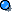Before Newton formulated his laws of motion and the law of universal gravitational attraction, German astronomer Johannes Kepler deduced from astronomical observations three laws (Kepler’s laws) that describe the motions of the planets.

### Acceleration in Orbit

Newton's first law describes how, once in motion, planets remain in motion. What it does not do is explain how the planets are observed to move in nearly circular orbits rather than straight lines. Enter the second law. To move in a curved path, a planet must have an acceleration toward the center of the circle. This is called centripetal acceleration and is supplied by the mutual gravitational attraction between the sun and the planet.

#### Motion in a Circular Orbit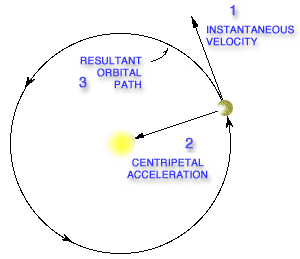### Kepler's Laws

Johannes Kepler's laws, as expressed by Newton, are:1. If two bodies interact gravitationally, each will describe an orbit that is a conic section about the common mass of the pair. If the bodies are permanently associated, their orbits will be ellipses. If they are not permanently associated with each other, their orbits will be hyperbolas (open curves).

2. If two bodies revolve around each other under the influence of a central force (whether or not in a closed elliptical orbit), a line joining them sweeps out equal areas in the orbital plane in equal intervals of time.

3. If two bodies revolve mutually about each other, the sum of their masses times the square of their period of mutual revolution is in proportion to the cube of the semi-major axis of the relative orbit of one about the other.

The major application of Kepler's first law is to precisely describe the geometric shape of an orbit: an ellipse, unless perturbed by other objects. Kepler's first law also informs us that if a comet, or other object, is observed to have a hyperbolic path, it will visit the sun only once, unless its encounter with a planet alters its trajectory again.

Kepler's second law addresses the velocity of an object in orbit. Conforming to this law, a comet with a highly elliptical orbit has a velocity at closest approach to the sun that is many times its velocity when farthest from the sun. Even so, the area of the orbital plane swept is still constant for any given period of time.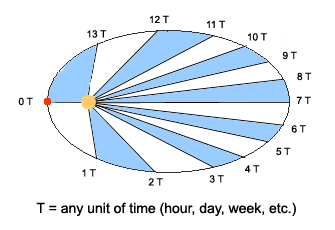CLICK IMAGE TO START / STOP ANIMATION

Kepler's third law describes the relationship between the masses of two objects mutually revolving around each other and the determination of orbital parameters. Consider a small star in orbit about a more massive one. Both stars actually revolve about a common center of mass, which is called the barycenter. This is true no matter what the size or mass of each of the objects involved. Measuring a star's motion about its barycenter with a massive planet is one method that has been used to discover planetary systems associated with distant stars.

Obviously, these statements apply to a two-dimensional picture of planetary motion, which is all that is needed for describing orbits. A three-dimensional picture of motion would include the path of the sun through space.

### Gravity Gradients & Tidal Forces

Gravity's strength is inversely proportional to the square of the objects' distance from each other. For an object in orbit about a planet, the parts of the object closer to the planet feel a slightly stronger gravitational attraction than do parts on the other side of the object. This is known as gravity gradient. It causes a slight torque to be applied to any orbiting mass which has asymmetric mass distribution (for example, is not spherical), until it assumes a stable attitude with the more massive parts pointing toward the planet. An object whose mass is distributed like a bowling pin would end up in an attitude with its more massive end pointing toward the planet, if all other forces were equal.

Consider the case of a fairly massive body such as our Moon in Earth orbit. The gravity gradient effect has caused the Moon, whose mass is unevenly distributed, to assume a stable rotational rate which keeps one face towards Earth at all times, like the bowling pin described above.

The Moon's gravitation acts upon the Earth's oceans and atmosphere, causing two bulges to form. The bulge on the side of Earth that faces the moon is caused by the proximity of the moon and its relatively stronger gravitational pull on that side. The bulge on the opposite side of Earth results from that side being attracted toward the moon less strongly than is the central part of Earth. Earth's atmosphere and crust are also affected to a smaller degree. Other factors, including Earth's rotation and surface roughness, complicate the tidal effect. On planets or satellites without oceans, the same forces apply, causing slight deformations in the body. This mechanical stress can translate into heat, as in the case of Jupiter's volcanic moon Io.

#### For Further Study

Select the "Links" section below for additional references, including mathematical tutorials and example problems.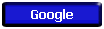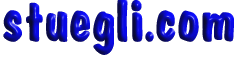Copyright © 2005 -  S. B. EglI